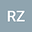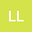On the Wolff-type Integral System with Negative Exponents
••Rong ZhangInstitute of Mathematics, School of Mathematics Science, Nanjing Normal University, Jiangsu Nanjing 210023, China

Corresponding Author:[email protected]

Author ProfileLing Li
Nanjing Normal University
Author Profile## Abstract

In this paper, we are concerned with the positive continuous entire solutions of the Wolff-type integral system \begin{equation*} \left\{ \begin{array}{ll} &u(x) =C_{1}(x)W_{\beta,\gamma} (v^{-q})(x), \\[3mm] &v(x) =C_{2}(x)W_{\beta,\gamma} (u^{-p})(x), \end{array} \right. \end{equation*} where $n\geq1$, $\min\{p,q\}>0$, $\gamma>1$, $\beta>0$ and $\beta\gamma\neq n$. In addition, $C_{i}(x) \ (i=1,2)$ are some double bounded functions. If $\beta\gamma\in (0,n)$, the Serrin-type condition is critical for existence of the positive solutions for some double bounded functions $C_{i}(x)$ $(i=1,2)$. Such an integral equation system is related to the study of the $\gamma$-Laplace system and $k$-Hessian system with negative exponents. Estimated by the integral of the Wolff type potential, we obtain the asymptotic rates and the integrability of positive solutions, and studied whether the radial solutions exist.